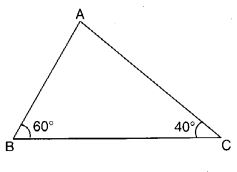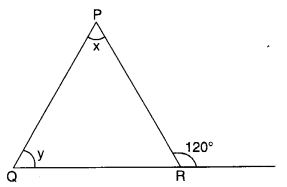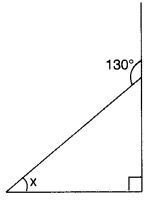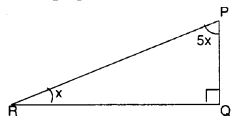# CBSEtips.in

## Thursday, 25 February 2021

### CBSE Class 7 Maths - MCQ and Online Tests - Unit 6 - The Triangles and its Properties

#### CBSE Class 7 Maths – MCQ and Online Tests – Unit 6 – The Triangles and its Properties

Every year CBSE students attend Annual Assessment exams for 6,7,8,9,11th standards. These exams are very competitive to all the students. So our website provides online tests for all the 6,7,8,9,11th standards’ subjects. These tests are also very effective and useful for those who preparing for any competitive exams like Olympiad etc. It can boost their preparation level and confidence level by attempting these chapter wise online tests.

These online tests are based on latest CBSE syllabus. While attempting these, our students can identify their weak lessons and continuously practice those lessons for attaining high marks. It also helps to revise the NCERT textbooks thoroughly

#### CBSE Class 7 Maths – MCQ and Online Tests – Unit 6 – The Triangles and its Properties

Question 1.
The measure of each angle of an equilateral triangle is
(a) 30°
(b) 45°
(c) 90°
(d) 60°

Hint:
x° + x° + x° = 180° ? x° = 60°.

Question 2.
Which of the following statements is true?
(a) A triangle can have two right angles
(b) A triangle can have two obtuse angles
(c) A triangle can have two acute angles
(d) A triangle can have all the three angles less than 60°

Answer: (c) A triangle can have two acute angles

Question 3.
How many angles are there in a triangle?
(a) 1
(b) 2
(c) 3
(d) 4

Hint:
See a triangle.

Question 4.
If two sides of a triangle are not equal, the triangle is called
(a) scalene
(b) isosceles
(c) equilateral
(d) right-angled

Hint:
Definition of a scalene triangle.

Question 5.
If two sides of a triangle are equal, the triangle is called
(a) isosceles
(b) equilateral
(c) scalene
(d) right-angled

Hint:
Definition of an isosceles triangle.

Question 6.
If all the three sides of a triangle are equal, the triangle is called
(a) equilateral
(b) right-angled
(c) isosceles
(d) scalene

Hint:
Definition of an equilateral triangle.

Question 7.
If all the angles of a triangle are acute, the triangle is called
(a) obtuse-angled
(b) acute-angled
(c) right-angled
(d) none of these

Hint:
Definition of an acute-angled triangle.

Question 8.
If one angle of a triangle measures 90°, the triangle is called
(a) acute-angled
(b) obtuse-angled
(c) right-angled
(d) none of these

Hint:
Definition of a right triangle.

Question 9.
If one angle of a triangle is obtuse, the triangle is called
(a) acute-angled
(b) obtuse-angled
(c) right-angled
(d) none of these

Hint:
Definition of an obtuse angled triangle.

Question 10.
How many medians can a triangle have?
(a) 1
(b) 2
(c) 3
(d) 4

Hint:
Draw medians and count.

Question 11.
How many altitudes can a triangle have?
(a) 1
(b) 2
(c) 3
(d) 4

Hint:
Draw altitudes and count.

Question 12.
The total measure of the three angles of a triangle is
(a) 360°
(b) 90°
(c) 180°
(d) none of these

Hint:
Angle Sum Property of a triangle.

Question 13.
How many elements are there in a triangle?
(a) 3
(b) 6
(c) 4
(d) None of these.

Hint:
See a triangle.

Question 14.
How many vertices does a triangle have?
(a) 1
(b) 2
(c) 3
(d) 4

Hint:
See a triangle.

Question 15.
How many sides are there in a triangle?
(a) 1
(b) 2
(c) 3
(d) 4

Hint:
See a triangle.

Question 16.
Two angles of a triangle measure 90° and 30°. The measure of the third angle is
(a) 90°
(b) 30°
(c) 60°
(d) 120°

Hint:
Third angle = 180° – (90° + 30°) = 60°.

Question 17.
The ratio of the measures of the three angles of a triangle is 2 : 3 : 4. The measure of the largest angle is
(a) 80°
(b) 60°
(c) 40°
(d) 180°

Hint:
Largest angle = $$\frac { 4 }{ 2+3+4 }$$ × 180° = 80°.

Question 18.
In the following figure, the side BC of ? ABC is extended up to the point D. If ?A = 55° and ?B = 60°, then the measure of ?ACD is(a) 120°
(b) 110°
(c) 115°
(d) 125°

Hint:
?ACD = 60° + 55° = 115°.

Question 19.
In the following figure, the measure of ?A is(a) 30°
(b) 45°
(c) 90°
(d) 30°

Hint:
?A – 180° – [(180° – 120°) + (180° – 120°)] = 60°.

Question 20.
In the following figure, the measure of ?A is(a) 70°
(b) 90°
(c) 80°
(d) 100°

Hint:
?A – 180° – (60° + 40°) = 80°

Question 21.
In the following figure, m || QR. Then, the measure of ?QPR is(a) 80°
(b) 85°
(c) 75°
(d) 70°

Hint:
?PQR = 50°
? ?QPR – 180° – (50° + 45°) = 85°.

Question 22.
In the following figure, find ? x and ? y, if ?x – ?y – 10°(a) 65°, 55°
(b) 55°, 45°
(c) 45°, 35°
(d) 60°, 60°

Answer: (a) 65°, 55°
Hint:
?x + ?y = 120°; ?x – ?y = 10° Solve to get ?x = 65°, ?y = 55°.

Question 23.
In the following figure, find ? B.(a) 30°
(b) 45°
(c) 40°
(d) 60°

Hint:
?B = 180° – [(180° – 110°) + 50°] = 60°.

Question 24.
Which of the following statements is true?
(a) A triangle can have all the three angles equal to 60°.
(b) A triangle can have all the three angles greater than 60°.
(c) The sum of any two angles of a triangle is always greater than the third angle.
(d) The difference between the lengths of any two sides of a triangle is greater than the length of the third side

Answer: (a) A triangle can have all the three angles equal to 60°.

Question 25.
Which of the following statement is false?
(a) The sum of the lengths of any two sides of a triangle is less than the third side.
(b) In a right-angled triangle, the square on the hypotenuse = sum of the squares on the legs.
(c) If the Pythagorean property holds, the triangle must be right-angled.
(d) The diagonal of a rectangle produce ‘by itself the same area as produced by its length and breadth

Answer: (a) The sum of the lengths of any two sides of a triangle is less than the third side.

Question 26.
In the following figure, find x if BA || CE.(a) 60°
(b) 40°
(c) 45°
(d) 65°

Hint:
?ECD = ?ABC = 50°;
? x = 180° – (65° + 50°) = 65°.

Question 27.
Find the value of the unknown interior angle x in the following figure:(a) 30°
(b) 35°
(c) 40°
(d) 45°

Hint:
x + 90° = 130° ? x = 40°.

Question 28.
Find the value of unknown x in the following figure:(a) 40°
(b) 50°
(c) 45°
(d) 55°

Hint:
x + 40° + 90° = 180° ? x = 50°.

Question 29.
Find the value of unknown x in the following figure:(a) 10°
(b) 15°
(c) 20°
(d) 25°

Hint:
x + 5x + 90° = 180° ? x = 15°.

Question 30.
Find angle x in the following figure:(a) 90°
(b) 80°
(c) 95°
(d) 100°

Hint:
x + 45 + 45° = 180° ? x = 90°.

Question 31.
In which case of the following lengths of sides of a triangle, is it possible to draw a triangle?
(?) 3 cm, 4 cm, 7 cm
(b) 2 cm, 3 cm, 7 cm
(c) 3 cm, 4 cm, 5 cm
(d) 3 cm, 3 cm, 7 cm

Answer: (c) 3 cm, 4 cm, 5 cm
Hint:
3 + 4 > 5; 4 + 5 > 3; 5 + 3 > 4.

Question 32.
Which of the following cannot be the sides of a right triangle?
(?) 2 cm, 2 cm, 4 cm
(b) 5 cm, 12 cm, 13 cm
(c) 6 cm, 8 cm, 10 cm
(d) 3 cm, 4 cm, 5 cm

Answer: (?) 2 cm, 2 cm, 4 cm
Hint:
2² + 2² = 8;
4² = 16
? 2² + 2² ? 4²

Question 33.
Find angle x in the following figure:(a) 40°
(b) 50°
(c) 45°
(d) 60°

Hint:
x = 50°.

Question 34.
Find angle x in the following figure:(a) 40°
(b) 30°
(c) 25°
(d) 35°

Hint:
x + x + 120° = 180° ? x = 30°.

Question 35.
Find angle x in the following figure:(a) 40°
(b) 45°
(c) 35°
(d) 50°

Hint:
x + 140° = 180° ? x = 40°.

Question 36.
Find angle x in the following figure:(a) 58°
(b) 59°
(c) 57°
(d) 56°

Hint:
x + x = 116° ? x = 58°.

Question 37.
Find angle x in the following figure:(a) 45°
(b) 40°
(c) 35°
(d) 50°

Hint:
x = 45°.

Question 38.
In the following figure, ? ABC is an equilateral triangle. Find ?x.(a) 30°
(b) 45°
(c) 60°
(d) 90°

Hint:
?ABC = 60°.
? ?ABD = 180° – 60° = 120°
? x = 180° – (120° + 30°) = 30°.

Question 39.
In the following figure, one angle of triangle ABC is 40°. If the difference of the other two angles is 30°, find the larger of the other two angles.(a) 85°
(b) 80°
(c) 75°
(d) 70°

Hint:
x + y = 180° – 40° = 140° x – y = 30°. Solve to get x = 85°.

Question 40.
In the following figure, find(a) 60°
(b) 70°
(c) 80°
(d) 75°

Hint:
x + x = 140° ? x = 70°.

Share: